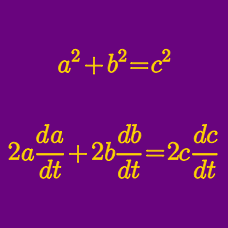Calculus

# Related Rates: Level 2 Challenges

If I throw a stone into a pond, then a ripple will emanate from the point of impact of the stone with the water's surface. As the ripple begins to spread outward, the radius of the ripple grows at 7 inches per second. How large will the circumference of the ripple be (in inches) after 5 seconds?

You and I are each holding one of the ends of a 27-foot rope that passes over a pulley. The distance from the pulley to a point on the ground directly under the pulley is 8 feet and you are standing 15 feet from that point. If I start walking away from you at 5 feet per second, then how fast are you being pulled in my direction, in feet per second?

Uh oh. Your ice cream has begun to melt! Liquefied ice cream begins to fill the bottom of your 6cm diameter, 10cm tall waffle cone at a rate of 2cm$$^3$$ per minute. How fast is the depth (in cm/min) of the melted ice cream increasing when the volume of the liquid ice cream is $$\frac{15}{4}\pi$$?A 6 foot tall painting is placed on the wall so that the very bottom of the painting sits 7 feet above the floor. Suppose your eyes are 5 feet above the floor and that you are standing 6 feet from the painting. Let T be your line of sight with the top of the painting and B be your line of sight with the bottom of the painting. What is the absolute value of the rate the angle formed by T and B is changing (in radians per second) as you approach the painting at 3 feet per second?

The amount of heat dissipated through a resistor of resistance $$R$$ through a circuit with voltage $$V$$ for $$t$$ seconds is approximated by the formula $$H = \displaystyle\frac{V^2t}{R}$$.

After the circuit has been on for 5 seconds, the resistor has been heating up and its resistance is $$10 \Omega$$ while increasing at a rate of $$1 \Omega / \text{s}$$. What is the rate (in J/s) that heat is being dissipated through this resistor at this moment if the circuit is operating at a constant voltage of $$V = 12$$?

×

Problem Loading...

Note Loading...

Set Loading...